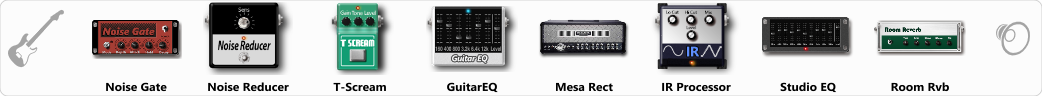# Master or Ride

Discussion in 'ToneLib-GFX presets' started by BlackTears, Feb 5, 2022.

1. Master or Ride

Preset name: Master of Puppets may be ride

Effects chain:Effect: "Noise Gate" (Dynamics / Filter), active - "yes"
"Detect" = Gtr In
"Mode" = Manual
"Depth" = 50
"Threshold" = 50
"Attack" = 0
"Hold" = 5
"Decay" = 250

Effect: "Noise Reducer" (Dynamics / Filter), active - "yes"
"Sens" = 72
"Mode" = Hard

Effect: "T-Scream" (Overdrive / Distortion), active - "yes"
"Drive" = 0
"Tone" = 100
"Level" = 61

Effect: "GuitarEQ" (Dynamics / Filter), active - "yes"
"160 Hz" = 2
"400 Hz" = 2
"800 Hz" = -1
"1.6 kHz" = 6
"3.2 kHz" = 15
"6.4 kHz" = -1
"12 kHz" = -15
"Level (dB)" = 0

Effect: "Mesa Rect" (Amp simulators), active - "yes"
"Gain" = 70
"Bass" = 87
"Middle" = 39
"Treble" = 76
"Presence" = 50
"Master" = 50
"Output" = 50
"Level (dB)" = 8

Effect: "IR Processor" (Dynamics / Filter), active - "yes"
"IR" = puppet_stereo_IR
"Low Cut (Hz)" = 0
"Hi Cut (kHz)" = 20.0
"Mix" = 100
"Level (dB)" = 0

Effect: "Studio EQ" (Dynamics / Filter), active - "yes"
"31 Hz" = 6
"62 Hz" = 4
"125 Hz" = 3
"250 Hz" = -2
"500 Hz" = -2
"1 kHz" = 1
"2 kHz" = 1
"4 kHz" = -3
"8 kHz" = 0
"16 kHz" = 0
"above 16 kHz" = 0
"Level (dB)" = 0

Effect: "Room Rvb" (Reverb), active - "yes"
"Time" = 4.0
"PreDelay" = 17
"LoDamp" = 100
"HiDamp" = 43
"Mix" = 20

Note: You will need to download and install the ToneLib-GFX software to use the preset.

File size:
1.7 KB
Views:
1,753